International
Tables for
Crystallography
Volume D
Physical properties of crystals
Edited by A. Authier

International Tables for Crystallography (2006). Vol. D, ch. 2.4, pp. 329-330

## Section 2.4.2.2. Piezoelectric media

R. Vachera* and E. Courtensa

aLaboratoire des Verres, Université Montpellier 2, Case 069, Place Eugène Bataillon, 34095 Montpellier CEDEX, France
Correspondence e-mail:  rene.vacher@ldv.univ-montp2.fr

#### 2.4.2.2. Piezoelectric media

| top | pdf |

In piezoelectric crystals, a stress component is also produced by the internal electric field E, so that the constitutive equation (2.4.2.2)has an additional term (see Section 1.1.5.2),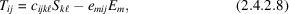where e is the piezoelectric tensor at constant strain.

The electrical displacement vector D, related to E by the dielectric tensor, also contains a contribution from the strain,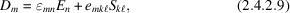whereis at the frequency of the elastic wave.

In the absence of free charges,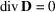, and (2.4.2.9)provides a relation between E and S,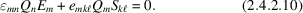For long waves, it can be shown that E and Q are parallel. (2.4.2.10)can then be solved for E, and this value is replaced in (2.4.2.8)to give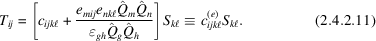Comparing (2.4.2.11)and (2.4.2.2), one sees that the effective elastic tensor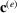now depends on the propagation direction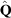. Otherwise, all considerations of the previous section, starting from (2.4.2.6), remain, with c simply replaced by.